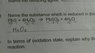# Redox reaction-naming the substance reduced

• wei1006
In summary, the substance reduced in the reaction is PbSO4. Relevants equations are-Reduction is the decrease in the oxidation state of an element in a substance during a reaction.-Oxidation state of lead in PbS varies but in this case I think should be +2-Oxidation state of S in PbSO4 is +6-Oxidation state of O in PbSO4 is -8, not really sure if it is -8 or -2 as my states that oxidation state of oxygen in compounds except peroxidea is fixed as -2 but shouldn't it multiply by 4 as it is PbSO4 and that makes sense because it is (SO4)2- thus I go with

#### wei1006

1) Problem statement:
Name the substance reduced in the reaction:
PbS + 4 H2O2 --> PbSO4 + 4H2O

Explain why the reaction is a redox reaction.

2) Relevent equations:
Not much equations...
-Reduction is the decrease in the oxidation state of an element in a substance during a reaction.
-
3) Attempt the question:

-Oxidation state of lead in PbS varies but in this case I think should be +2
-Oxidation state of Sulfur in PbS is -2
-Oxidation state of Hydrogen in H2O2 is +2, not really sure if it is +2 or +1 as my states that oxidation state of hydrogen in compounds except metal hydride is fixed as +1 but shouldn't it multiply by 2 as it is H2O2?
-Oxidation state of oxygen in H2O2 is +2, not really sure if it is +1 or +2 as my states that oxidation state of oxygen in peroxides such as H2O2 is fixed as +1 but shouldn't it multiply by 2 as it is H2O2?
-Oxidation state of lead in PbSO4 varies but in this case I think should be +2
-Oxidation state of S in PbSO4 is +6
-Oxidation state of O in PbSO4 is -8, not really sure if it is -8 or -2 as my states that oxidation state of oxygen in compounds except peroxidea is fixed as -2 but shouldn't it multiply by 4 as it is PbSO4 and that makes sense because it is (SO4)2- thus I go with -8?
-Oxidation state of hydrogen in H2O2 is +2, not really sure if it is +2 or +1 as my states that oxidation state of hydrogen in compounds except metal hydride is fixed as +1 but shouldn't it multiply by 2 as it is H2O2?
-Oxidation state of oxygen in H2O is -2

So I have compiled it into the following picture(attachment) for clearer viewing, I have written oxidation state for respective elements below the reaction equation.

But since the definition given is: reduction is the decrease in oxidation state in the element, I don't really understand how does that work in a chemical equation, thus not able to come out with an answer. Does that mean to add up all oxidation state of all elements in the substance to find out with one decrease before and after? But in that case, all will be 0...

For the explanation part, I couldn't identify the substance reduced so I couldn't answer yet.

#### Attachments

•1419939482017.jpg
31.9 KB · Views: 680
wei1006 said:
lead in PbS varies but in this case I think should be +2
It does NOT vary.
wei1006 said:
Sulfur in PbS is -2
Correct.
wei1006 said:
hydrogen in compounds except metal hydride is fixed as +1 but shouldn't it multiply by 2 as it is H2O2?
You do NOT multiply by stoichiometry.
wei1006 said:
oxidation state of oxygen in peroxides such as H2O2 is fixed as +1 but shouldn't it multiply by 2 as it is H2O2?
You do NOT multiply by stoichiometry.
Now, try it with the single clarification that you do NOT multiply by stoichiometry.

•wei1006
In other words - oxidation state is a property of an atom, not of all atoms of a given element in the molecule combined. It is quite possible to have two atoms of the same element in a single molecule, each with a different oxidation state (which is not the case in substances listed in this problem).

•wei1006
The oxidation state of an element in a compound is given for one of its atoms, so you don't have to multiply it by the number of atoms actually present in the compound. For example, Na2O and Al2O3 have different number of oxygen atoms in each compound, but oxygen has the same oxidation number(-2) in both cases, even though there are 3 oxygen atoms in aluminum oxide(its oxidation state is NOT -2 x 3). With this logic, try working out the oxidation numbers for each atom in the original question on both sides of the equation.

•wei1006
Thank you all for the replies! Have cleared my misconception and worked out the problem :)